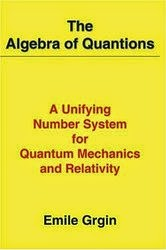## Quantionic Quantum Mechanics

In the prior posts I presented the alternatives to complex quantum mechanics: real, and quaternionic quantum mechanics. it turned out that real and quaternionic quantum mechanics are constrained versions of complex quantum mechanics and as such they have several problems: lack of a tensor product (because the constraints do not scale  nicely with the tensor product), lack of a de Finetti representation, etc. Are there other possible number systems for quantum mechanics?

If quantum mechanics predicts only probabilities, there would be no other possibility and Adler proves why in his quaternionic quantum mechanics monograph. But this is absurd! How can quantum mechanics predict something other than probabilities? How about generalizing probabilities to probability current densities? This is actually what one gets in Dirac's theory of the electron! It turns out that there exists a completely equivalent formulation of Dirac theory of spinors in terms of a new quantum mechanics number system called the quantions and this would correspond to a different factorization of the d'Alembertian. This was discovered by Emile Grgin and I will expose this beautiful theory in this and subsequent posts.Grgin's quantions book

So let us start from the beginning. The algebraic structure of quantum mechanics corresponds to two algebras: the Jordan algebra of the observables, the Lie algebra of the generators, and their compatibility relationship (see definition 2.1 on page 7 on this).

Now it turns out that the compatibility relationship allows the introduction of an associative product which in the case of complex quantum mechanics is the usual complex number multiplication. Associativity has an experimental interpretation: the ability to compose two experiments sequentially.

The map J ($$J^2 = -1$$) between observable and generators can be introduced in two ways:
• the usual way as sqrt(-1)
• an internal way as an element of the algebra of observables
In the second case let this special element be: $$O_J$$ and to preserve invariance under tensor composition we demand that all other observables must commute with $$O_J$$ So what we get is a sub-algebra of the two-product Jordan-Lie algebra and now we have a constained quantum mechanics compatible with the tensor product (unlike quaternionic quantum mechanics). In fact this is the BRST theory in disguse.

Now if we want to find something others than complex number quantum mechanics we need to look at non-unitary representations. This means that the associative product is no longer a division number system and hence we can get non-negative probabilities or "ghosts". But isn't this nonphysical?  Sure it is. However restricting the observables to be only the ones commuting with $$O_J$$ restores sanity. The only price to pay is the generalization of the inner product.

It turns out that the unphysical quantum mechanics corresponds to quantum mechanics over $$SO(2,4)$$ which is isomorphic with $$SU(2,2)$$ and this contains two positive probability modes and two negative probability modes. However, restricting the observable to commute with $$O_J$$ restores positivity and what results is a non-division algebra $$SL(2,C)\oplus SL(2,C)$$ which Grgin calls the quantionic algebra.

Quantionic quantum mechanics is actually the gauge theory of electroweak interaction, is a BRST theory, corresponds to a Hodge decomposition of the d'Alembertian, and is fully equivalent with the spinor Dirac theory. All we have to accept is the fact that experiments do not result in probabilities but in the natural generalization of them in the special theory of relativity context: Dirac's current probability densities. Quantionic quantum mechanics is the natural unification of quantum mechanics and relativity. Moreover, this can only work in 3+1 spacetime dimensions! Spin, theory of relativity, and space-time dimensionality emerge naturally in this theory and they do not have to be assumedWe'll explore in depth this theory in the next posts.